#Function Repository Resource:

# GenerateTiling

Generate a tiling pattern from a set of local template constraints

Contributed by: Wolfram Research
 ResourceFunction["GenerateTiling"][templates,{},n] covers an n×n array with values consistent with local constraints listed in templates. ResourceFunction["GenerateTiling"][templates,{},{m,n}] covers an m×n array instead. ResourceFunction["GenerateTiling"][templates,{},size,All] lists all array coverings consistent with local constraints listed in templates. ResourceFunction["GenerateTiling"][templates,{},size,count] lists multiple coverings, up to integer count of them. ResourceFunction["GenerateTiling"][templates,seed,size,count] forces the seed array to occur near the center of the pattern.

## Details

A tiling pattern is any rectangular array of non-negative integers.
A template is a rectangular array containing non-negative integers or Blank (_) values.
A Blank value in a template can match any integer in a tiling pattern.
For example, the template {{_,0,2},{0,1,_}} has four different integer values and two Blank (_) values.
ResourceFunction["GenerateTiling"] assumes that possible values in a tiling pattern are bounded above by the maximum integer found in the set of templates.
Sets of potentially many templates should have the same bounding size, say {a,b}.
If all {a,b} subarrays of an {m,n} pattern match a template from input templates, the tiling pattern is said to be "consistent with local constraints listed in templates", or for short, "template consistent".
Values on the edge of a pattern should be checked against partial templates by allowing complete templates to partially intersect the tiling pattern and ignoring the overhang to exterior.
ResourceFunction["GenerateTiling"] takes the option "Boundary". When set to "Periodic", ResourceFunction["GenerateTiling"] also requires template consistency of a tiling pattern across North-South and East-West edges (i.e. toroidal boundary conditions).

## Examples

### Basic Examples (4)

Generate a uniform tiling pattern of all 0s:

 In:=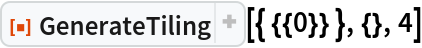Out=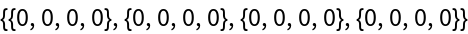Generate uniform tiling patterns for the first few positive integers:

 In:=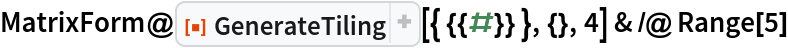Out=Generate a tiling pattern from a set of three templates:

 In:=Out=Plot the tiling pattern using ArrayPlot, and use the resource function TilingPatternPlot to show the templates used:

 In:=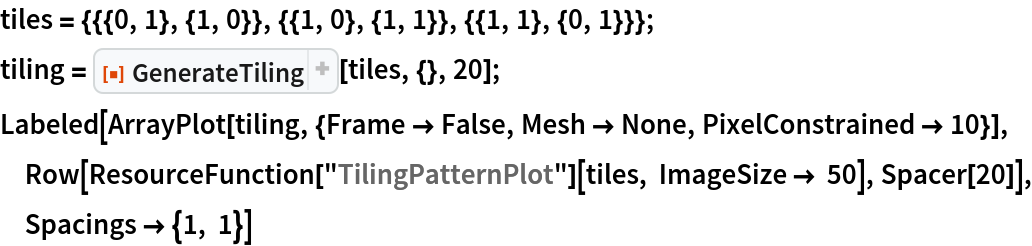Out=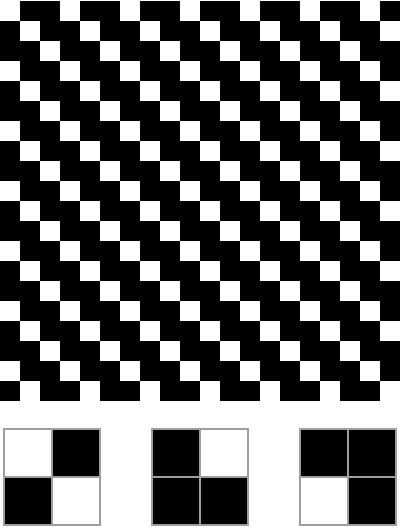Change the dimensions of the tiling pattern and break up the result into generating tiles:

 In:=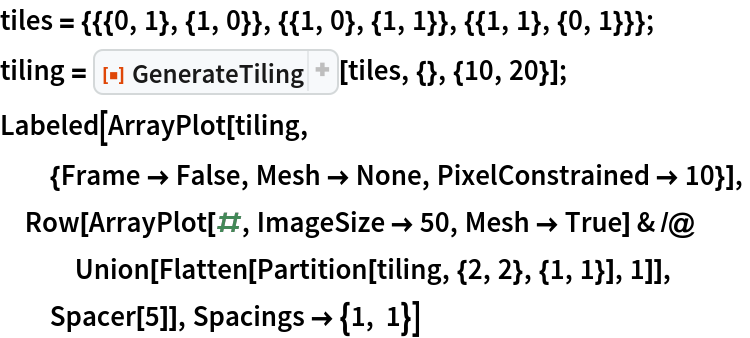Out=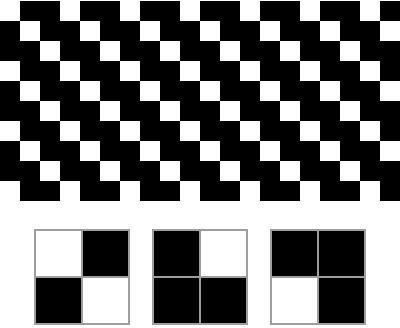Plot a four-color periodic tiling pattern:

 In:=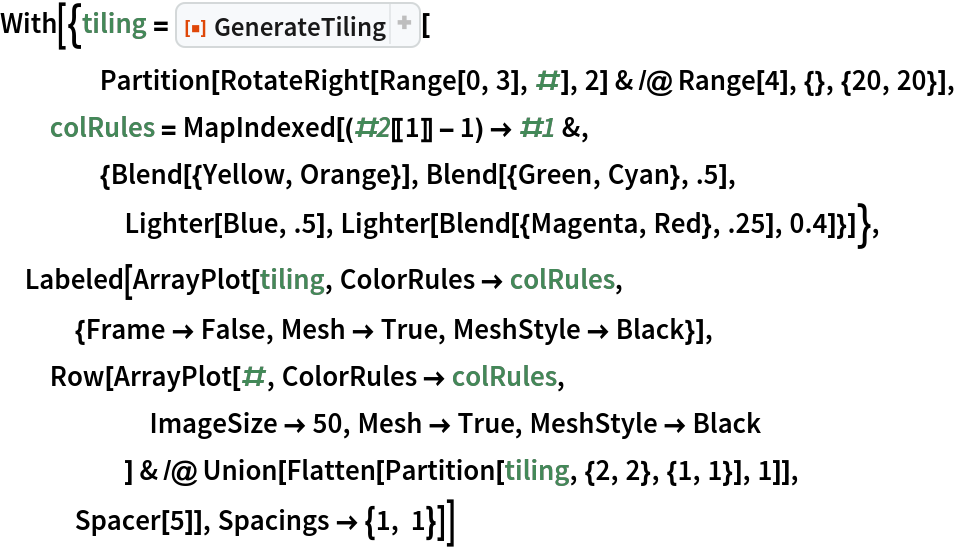Out=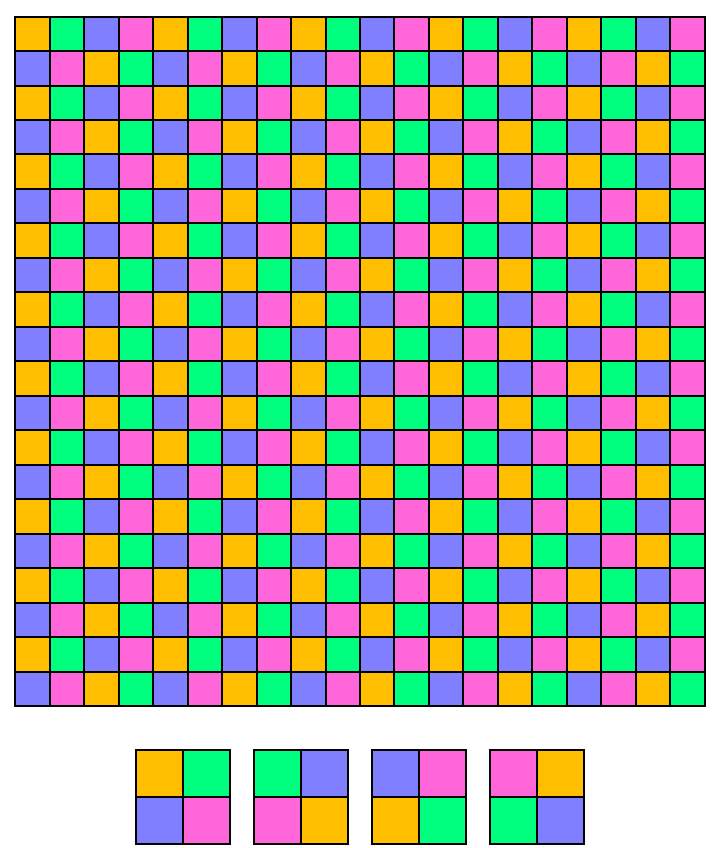Plot all four translates of this tiling pattern:

 In:=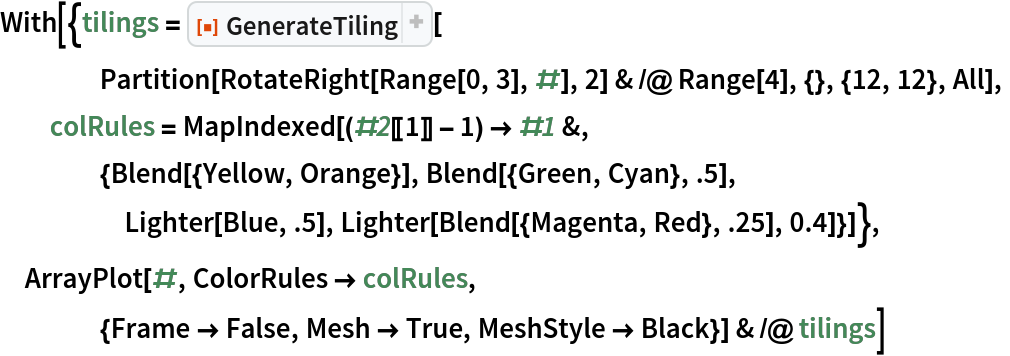Out=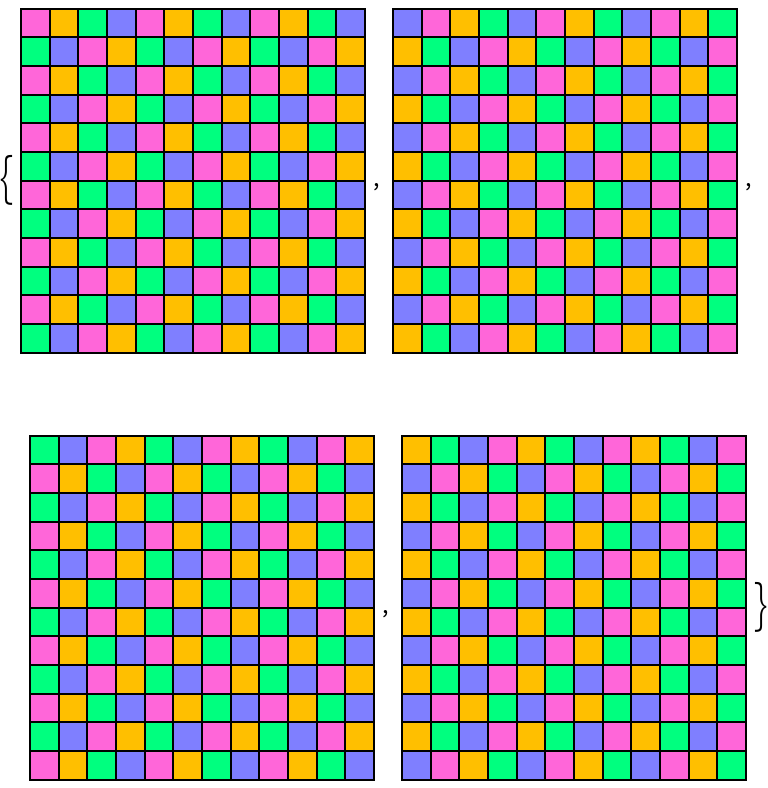### Scope (2)

Generate a tiling pattern from a complicated set of templates:

 In:=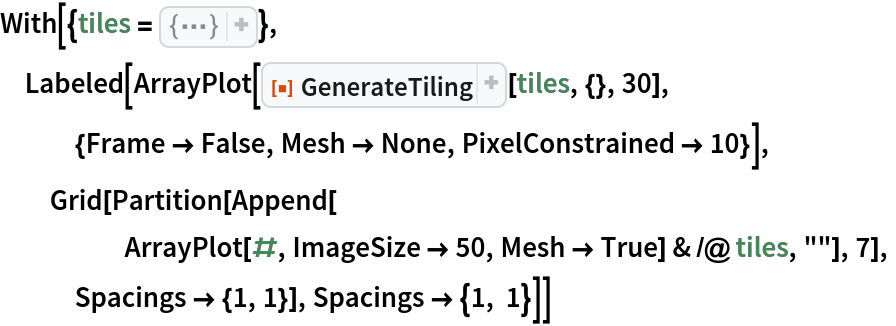Out=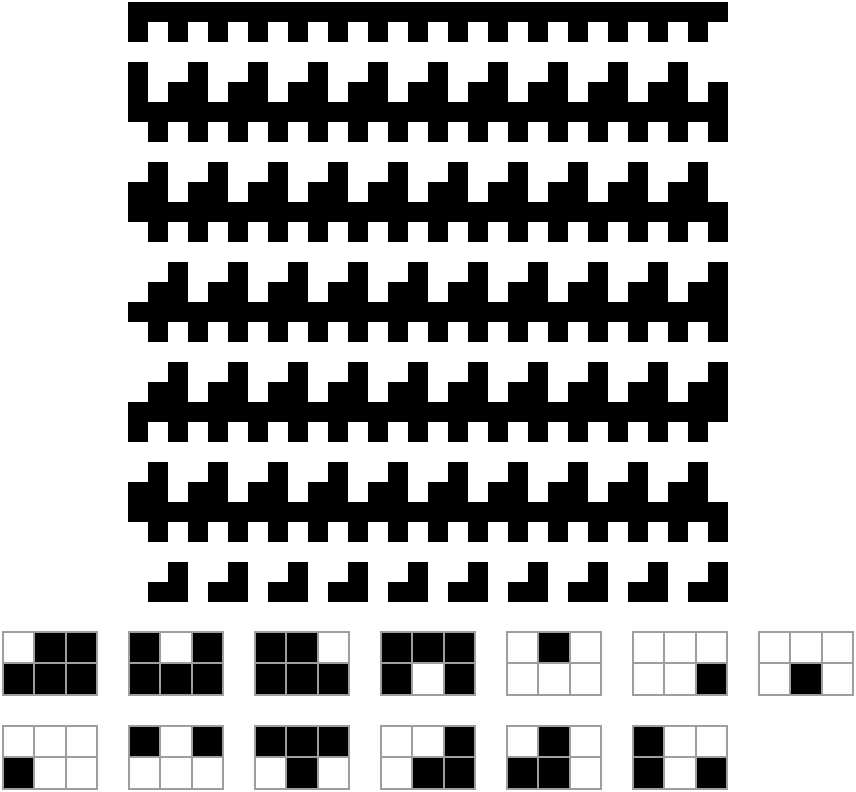Control the offset of a periodic tiling pattern by setting a seed value:

 In:=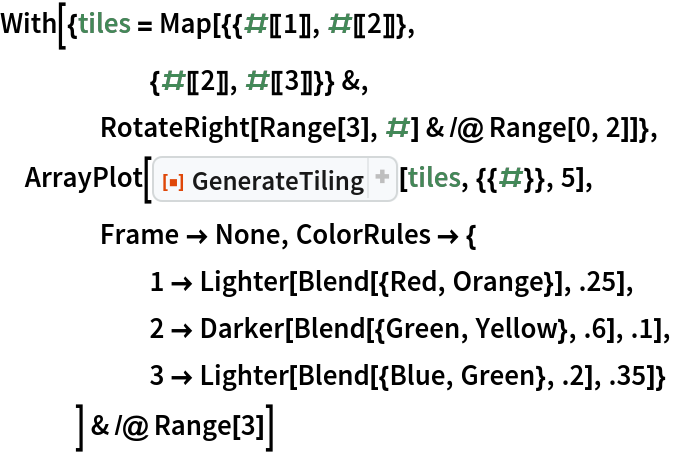Out=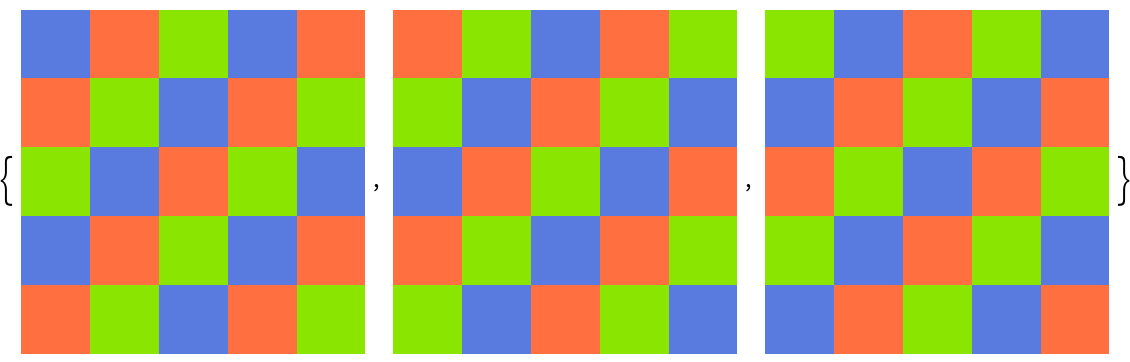A tiling pattern around an {{n,n}} initial condition is not possible for this set of tiles:

 In:=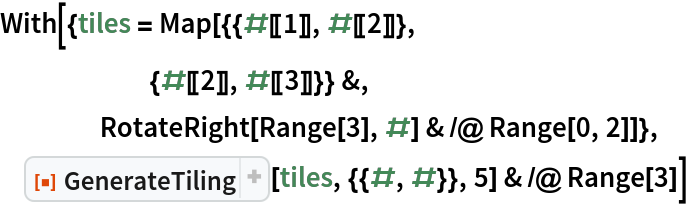Out=### Options (3)

#### Boundary (3)

Generate a tiling on a size-30 torus (i.e. a tiling with a size 30×30 periodic boundary):

 In:=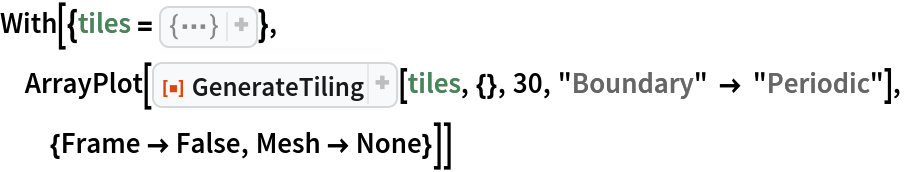Out=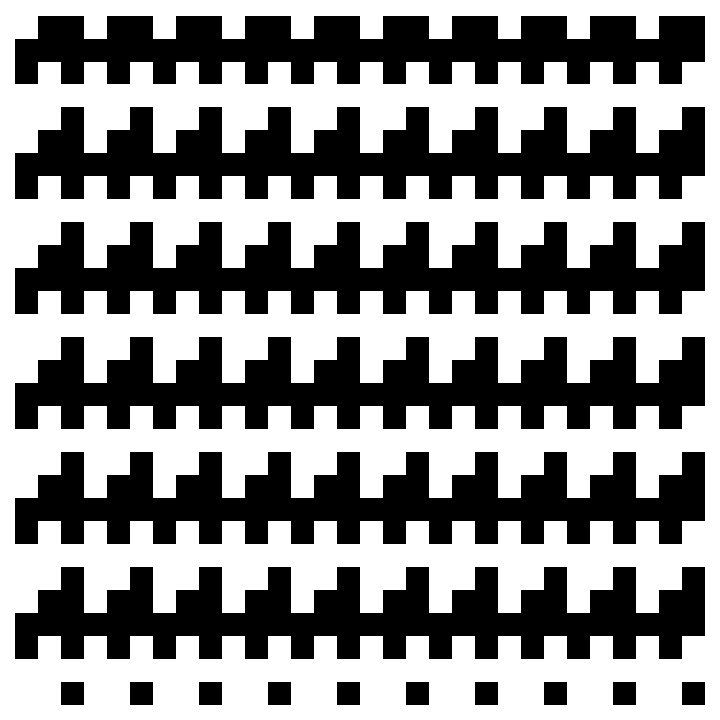The minimum periodic region has size 5×6:

 In:=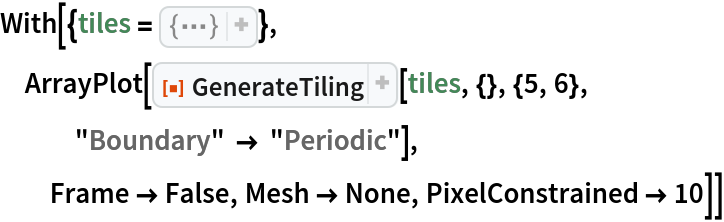Out=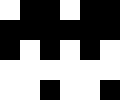Double-check that value by counting inequivalent translates:

 In:=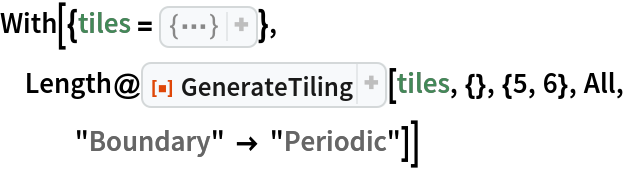Out=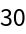### Possible Issues (2)

Templates must have the same bounding dimensions:

 In:=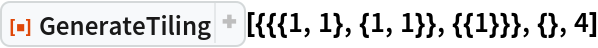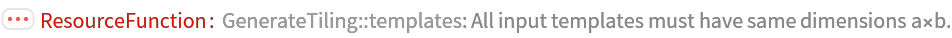Out=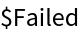If not, it is always possible to add more blanks:

 In:=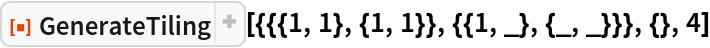Out=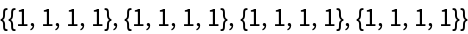### Neat Examples (2)

Generate a periodic tiling pattern from a complicated set of tiles:

 In:=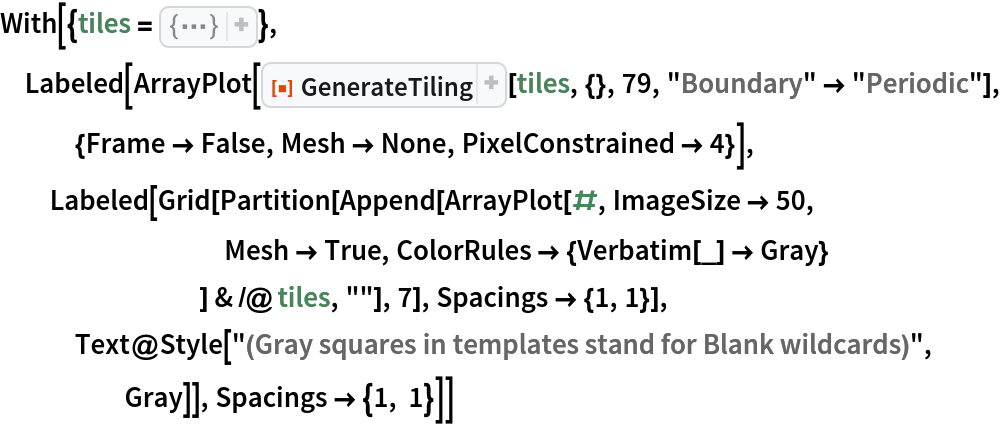Out=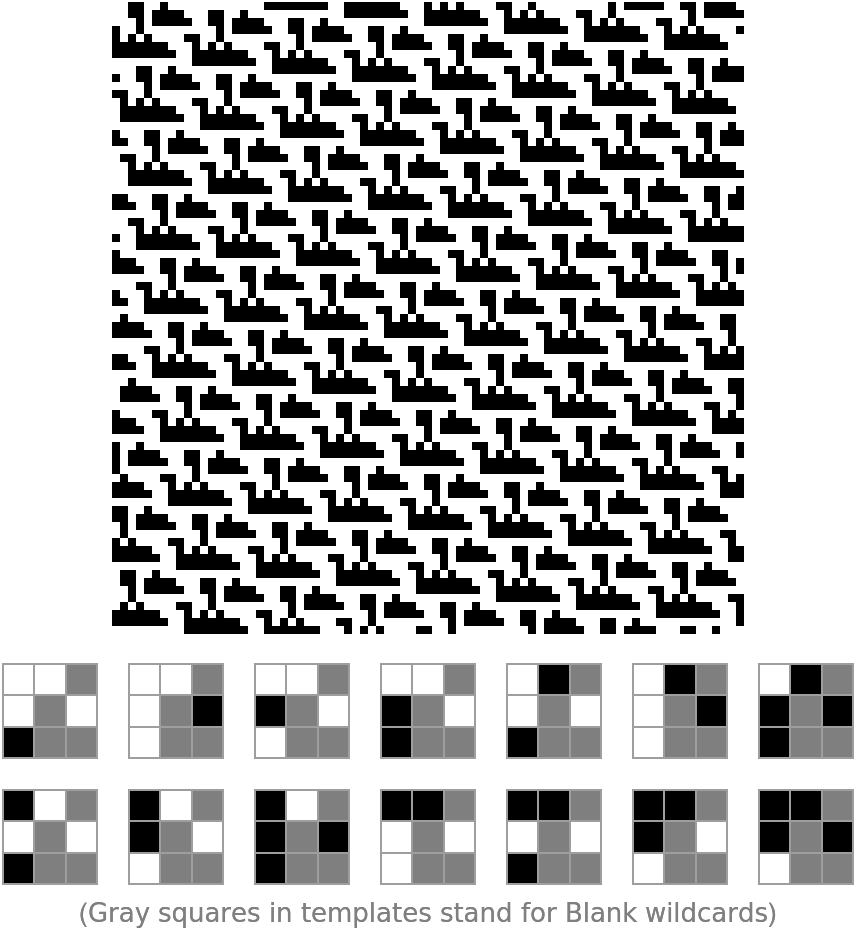Generate the nested pattern from NKS, Chapter 5 (p. 219):

 In:=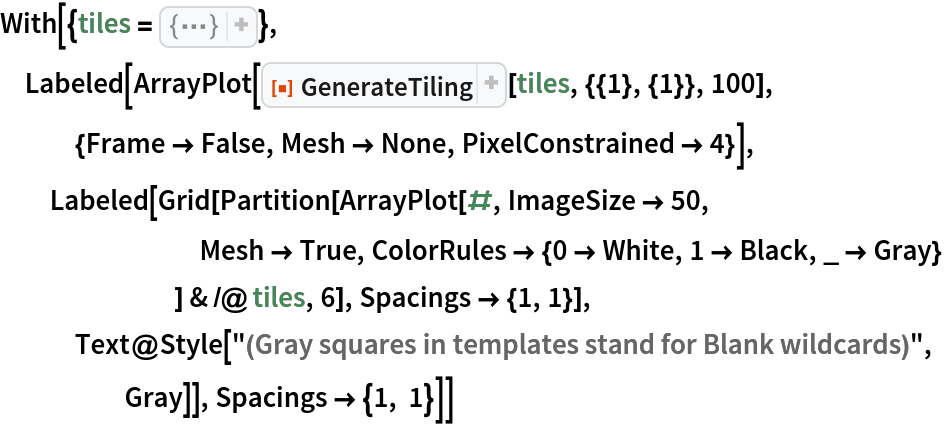Out=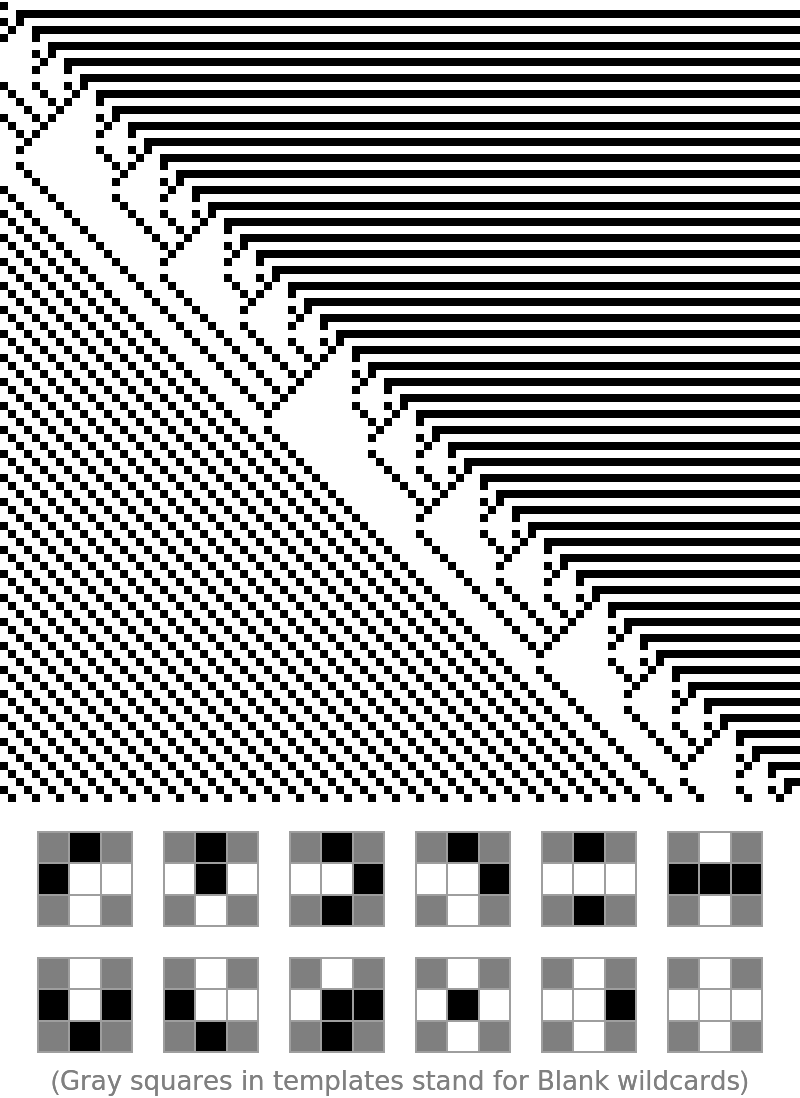## Version History

• 2.0.0 – 23 June 2022
• 1.0.0 – 25 March 2022

## Author Notes

Significant consistent revision with extended functionality. --Bradley Klee